# Entering formulas in worksheet cells

Use formulas in both spreadsheet and database cells to evaluate and perform operations on drawing data. Formulas always begin with an equal sign (=) and consist of a combination of functions, cell references, or operators that combine values to produce a new value, or a field identifier to select data attached to an object.

There are three ways to enter a formula in a cell:

Manually enter a formula in the Formula bar.

Use the Insert > Function and Insert > Criteria commands; see "Inserting a function" below.

For a database header row cell, click the button and select a field or function from the Set Function/Field dialog box; see Selecting a function or field for a database column.

To manually enter a formula:

Select the cell.

Enter an equal sign (=), and then enter the formula in the worksheet Formula bar. A formula can consist of functions, data field identifiers, operators, cell references, and/or constant values. As you type, a list of options that match the text displays; double-click an item to select it.

When the formula is complete, click the green check mark or press Enter to validate the entry. To cancel an entry, click the red X or press Esc.

The formula executes as soon as the cell entry has been validated (Auto-recalc must be selected in the worksheet preferences; see Worksheet menu).

## Inserting a function

 Command Path Function Insert (worksheet menu)

To enter a formula with the Function and Criteria commands:

Select the cell.

Enter an equal sign (=). The entry automatically displays in the worksheet Formula bar.

Select the command, and then select the desired field or function from The Select Function dialog box.

The formula is placed in the worksheet Formula bar.

Do one of the following:

If the function requires numbers or text, enter the argument between the parentheses and proceed to step 7.

If the function requires selection criteria, select Insert > Criteria from the Worksheet menu.

If an object is selected when the Criteria command is selected, the Paste Attributes dialog box opens. Otherwise, proceed to step 6.

Do one of the following:

To use attributes of the selected object as the only selection criteria, select the attributes and proceed to step 7.

To specify other criteria, or to use attributes of other objects in the drawing, click the Custom button.

The Criteria dialog box opens. Specify the criteria for the function.

When the formula is complete, click the green check mark or press Enter to validate the entry. To cancel the entry, click the red X or press Esc.

The formula executes as soon as the cell entry has been validated (Auto-recalc must be selected in the worksheet preferences; see Worksheet menu).

## Formula syntax

Formulas must be entered with a specific syntax. If the formula is not entered correctly, the formula entry itself displays in the cell, instead of the result of the formula. Two common mistakes in syntax include forgetting to use pairs of parentheses, and omitting required commas when arguments are present. Formula syntax is described in the following table.

Click to show/hide the syntax operators.Click to show/hide the syntax operators.

 Symbol Explanation Example General syntax Equal sign = Begins each formula; also indicates a value for a variable =CriteriaVolume(t=wall) Parentheses ( ) Encloses a function argument; also used in arithmetic equations =acos(0.6) =A6+(A6*.07) Square brackets [ ] Encloses a record destination =R IN ['myformat'] Period . Separates a record identifier and a field identifier =Furniture.Type Colon : Separates path name levels in cell references =MyWorksheet:A1 Comma or semicolon , or ; Separates multiple values in a function argument; use a semicolon when commas are used as decimal separators by the operating system =sum(A2,E3) =sum(A2;E3) Single quote ' Encloses a string constant =Appliances.'Model #' Dollar sign \$ Designates an absolute reference =A4*\$B\$1 Double period .. Designates a range of cells =sum(A10..A12) Arithmetic operators Plus sign + Addition =A6+A8 Hyphen - Subtraction =A6-A8 Asterisk * Multiplication =A6*.06 Forward slash / Division =B3/12 Caret ^ Exponentiation =13^2 DIV Integer division (returns the integer quotient of the division operation) j:= 36 DIV 5; MOD Remainder division (returns the remainder of the division operation as an integer) k:= 36 MOD 5; Comparison operators (used with IF function) Equal sign = Equal =if((L='L2'),Area,0) Less than and greater than signs (or Option+ = on Mac) <> or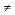Not equal =if((S<>'Dryer'),B9,0) Less than sign < Less than =if((C7<100),100,C7) Less than and equal signs (or Option+ < on Mac) <= or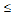Less than or equal to =if((E2<=G2),0.05,G2) Greater than sign > Greater than =if((C7>100),100,C7) Greater than and equal signs (or Option+ > on Mac) >= or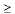Greater than or equal to =if((E2>=G2),0.05,G2)

To force the program to treat a number as text, enclose the number in single quotation marks, as in '40'; or format the cell as Text on the Number tab of the Format Cells dialog box.

Formulas follow standard algebraic rules of hierarchy. In the following example, the value in cell C28 is first multiplied by 12, and then 4.5 is subtracted from that value. The result is then divided by 12.

=((C28*12)-4.5)/12

There are several built-in functions that can be used in formulas, including mathematical functions and functions that pull information from objects in the drawing. To use one or more worksheet functions in a formula, either enter the function manually, or use the Insert > Function and Insert > Criteria commands to select a function and selection criteria (if required) from dialog boxes. See Worksheet functions for more information about how to use functions.

If there is a logic problem or calculation error with a formula, an error code displays in the cell.

Click to show/hide the error codes.Click to show/hide the error codes.

 Error Code Explanation #NAME? The field name in a database header row definition does not exist in the file; see Selecting a function or field for a database column #VALUE! The argument is the wrong type of data for the formula; for example, a cell referenced in a mathematical formula contains text #CVAL? A cyclical reference cannot be resolved ?Result? The result value type is unrecognized #OPCODE? Internal error #DIV 0! Division by zero error encountered #FAC? Unrecognized entry #OBJ! The worksheet name in an external reference does not exist, or the record name does not exist #CSTATUS? Functions are nested too deeply (more than ten levels deep)

## Referencing other worksheet cells

In a worksheet, a formula can reference the contents of one or more other cells. The cells can be referenced within the current worksheet (internal references), or from another worksheet (external references) within the same file.

External references must include the full path name to the other worksheet. The following table shows the syntax for entering an external reference into a formula.

If the name of the worksheet contains spaces, the name must be enclosed with single quotes as in the following example: ='Appliance Schedule':A1

To update an external reference, select File > Recalculate from the Worksheet menu.

### Relative and absolute cell references

Cell references in a worksheet can be either relative or absolute. When the formula that contains the reference is moved, an absolute reference always refers to the original cell address, while a relative reference changes depending on the location of the cell that contains the reference.

Use the dollar sign (\$) character to indicate an absolute reference. The \$ character locks the part of the cell reference it precedes, as described in the following table.

 Combination Description \$A1 Locks the specified column reference but leaves the row reference relative; the same column is always referred to, but the row changes if the formula is placed in a different row \$A\$1 Locks both the specified column and row references; regardless of where the formula is copied, it always refers to the original cell A\$1 Locks the specified row reference but leaves the column reference relative; the same row is always referred to, but the column changes if the formula is placed in a different column

In the following example worksheet, the formula =AVERAGE(B1..B3) is in cell B4. If the formula were copied to cell E9, the formula would automatically be changed to =AVERAGE(E6..E8). Because the references are relative, both the column and row would change relative to the cell where the formula is placed—always indicating the three cells directly above the formula.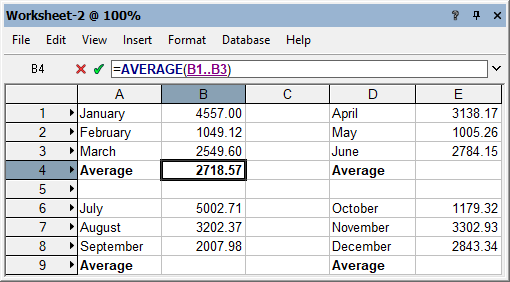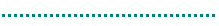Entering constant values in worksheet cells

Inserting images in worksheet cells

Worksheet functions